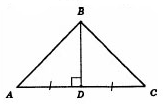# Answer to Question #6279 in Geometry for Elizabeth Mobley

Question #6279
If the legs of a triangle are 7 cm and the base is 5 cm, what is the area?
1
2012-02-03T07:58:54-0500
Consider triangle ABC:We know that AB=BC=7 cm, AC=5cm.
Let&#039;s draw height BD. As long as AC=BC , the height BD is also a median: AD=DC=AC/2=2.5
Consider triangle BDC, its right angled. Let&#039;s find BD using Pethagorean theorem:
BD^2+DC^2=BC^2
Hence BD=sqrt(BC^2-DC^2)=sqrt(7^2-2.5^2)= approx. 6.54
Now we can find area:
S=1/2*AC*BD=1/2*5*6.54=16,35 cm2

Need a fast expert's response?

Submit order

and get a quick answer at the best price

for any assignment or question with DETAILED EXPLANATIONS!